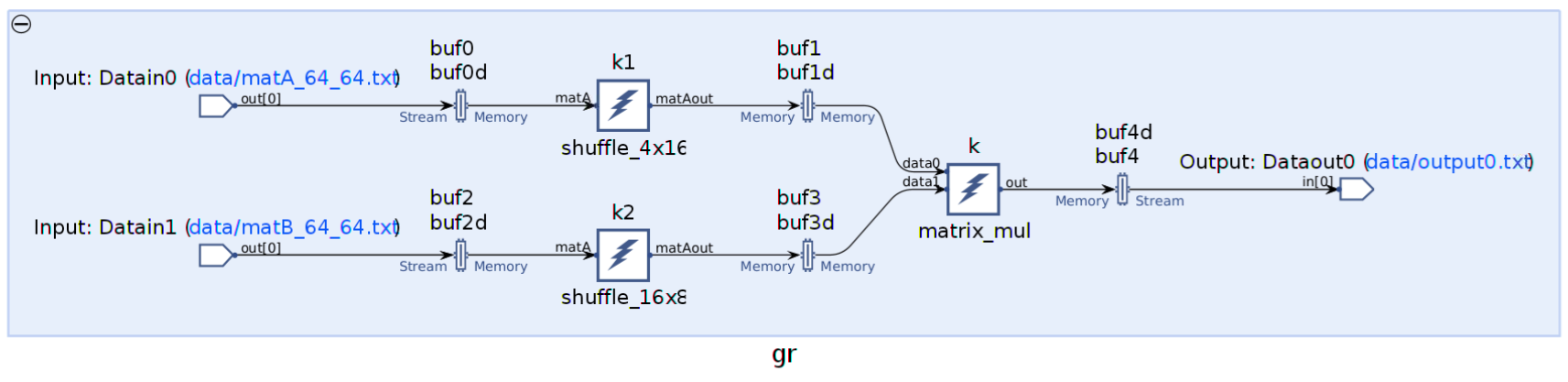# Matrix Multiplication - 2022.1 English

## AI Engine Kernel Coding Best Practices Guide (UG1079)

Document ID
UG1079
Release Date
2022-05-25
Version
2022.1 English

The AI Engine API provides a `aie::mmul` class template for a vector-based matrix multiplication. Multiple intermediate matrix multiplication results are accumulated to give the final result. For more details on the supported matrix multiplication shapes (`M*K*N`) and data types, see Matrix Multiplication .

The `aie::mmul` operations `mul` and `mac` accept row based data for the vector-based matrix multiplication. Then for the `mac` operation, arrange the data by `M*K` or `K*N` for better performance. This data shuffling can be done either in PL or AI Engine.

This section gives an example of ```(64 * 64) x (64 * 64)``` dimensions matrix multiplication. The data type is `int8 x int8`. The matrix multiplication shape `4*16*8` is chosen.

The input data is assumed to be row based. The data is input to the matrix multiplication kernel as `4*16` matrix and `16*8` matrix. Prior to the matrix multiplication kernel, the input data is shuffled.

The picture below shows the graph.

Figure 1. Matrix Multiplication Kernel Graph